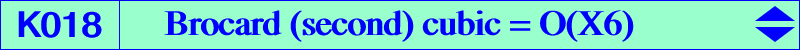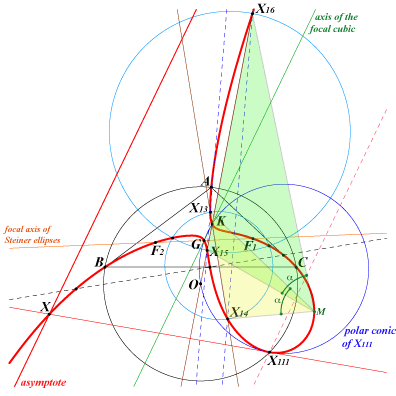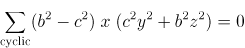X(2), X(6), X(13), X(14), X(15), X(16), X(111), X(368), X(524), X(5000), X(5001), X(11537), X(11549), X(32618), X(32619), X(39162), X(39163), X(39164), X(39165), X(47072), X(47073) isogonal conjugate of X(368) vertices of the second and fourth Brocard triangles real foci F1, F2 of the inscribed Steiner ellipse. foci of the K-ellipse (inellipse with center K when the triangle ABC is acute angle) other points below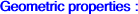K018 is a circular isogonal focal nK with root X(523) and singular focus X(111). The real asymptote is the homothetic of the line GK under h(X111, 2). K018 is also the orthopivotal cubic O(X6) and Z+(L) with L = X(3)X(6) in TCCT p.241. See also Z+(O) = CL025 and CL034. K018 is spK(X524, X2) or spK(X524, X6) in CL055, see also Table 48 and Table 55. It is a member of the class CL061. See Table 52 (Brocard-Grebe pencil) and another pencil in K1156. Locus properties Locus of point M such that the directed line angles (MX13, MX14) and (MX16, MX15) or (MX13, MX16) and (MX14,MX15) are equal (mod. π). In other words, the Fermat and isodynamic points are seen from M under equal or supplementary angles. See the related K508 and also K909. Locus of contacts of tangents drawn from X(111) to the circles passing through G and K. Locus of foci of inscribed conics whose center lies on the line GK, hence the cubic passes through the foci of the inscribed Steiner ellipse and also those of the inscribed conic with center K. Locus of point M such that the pole of the line MM* in the circum-conic through M and M* lies on the Brocard axis OK. Locus of point M such that K, M and the orthocorrespondent of M are collinear. Locus of point M such that the three circles MBC, MCA, MAB meet the sidelines of triangle ABC again at six points lying on a same conic. (equivalently) Locus of point M such that if the circle MBC meets AB, AC again at Ab, Ac and if A' = BAc /\ CAb (B', C' similarly) then ABC and A'B'C' are perspective. The perspector also lies on the curve. Since the line AA' contains the pole A" of BC in the circle MBC, another formulation is the following : the poles A", B", C" of BC, CA, AB in the circles MBC, MCA, MAB form a triangle perspective with ABC if and only if M lies on K018. Locus of point M such that if MB, MC meets the Kiepert hyperbola again at B', C' then the lines AP*, B'C', OK are concurrent. The cevian lines of point P meet the perpendicular bisectors of ABC at A', B', C'. The locus of P such that the sum of oriented lines angles (BA',BC)+(CB',CA)+(AC',AB) = 0 (mod. pi) is K018. When this sum is pi/2 (mod. pi), we obtain the Napoleon cubic. (Jean-Pierre Ehrmann) Locus of point M such that M and M* are conjugated with respect to the Kiepert hyperbola. See also Isogonal nK0 cubics and table 4. For any point P, the locus of the perspectors of the equilateral triangles centered at P which are perspective with ABC is the orthopivotal cubic O(P). When P = K, we find K018. Let P be a point and Pa, Pb, Pc its inversive images in the Apollonian circles C_a, C_b, C_c respectively. ABC and PaPbPc are perspective if and only if P lies on the circumcircle or on the Apollonius quartic Q049. The inversive image of Q049 in the circumcircle is K018. Let P be a point. The circles (Ba) and (Ca) pass through P and are tangent at B, C to AB and AC respectively. The circles (Cb), (Ab), (Ac) and (Bc) are defined cyclically. Then the points Ba, Ca, Cb, Ab, Ac and Bc lie on a same conic if and only if P lies on K018 (Angel Montesdeoca, 2016-04-28). Locus of point M such that X(6), M, Psi(M) are collinear. See Table 60. With the notations of 13, let A' be the intersection of BC with the radical axis of (Ba), (Ca) and define B', C' likewise. ABC and A'B'C' are perspective (at Q) if and only if P lies on the circumcircle (in which case X99 lies on the line PQ and Q lies on the Steiner ellipse) or P lies on K018 (in which case X2 lies on the line PQ and Q lies on K185). Angel Montesdeoca, 2017-06-01. Let ABC be a triangle and P be a point. Let Oa be the circumcenter of PBC and define Ob, Oc cyclically. Let S be the symmedian point of OaObOc.The locus of point P such that S lies on the Euler line of ABC is K018. (Kadir Altintas). More generally, S lies on a line (L) if and only if P lies on a bicircular quintic which decomposes into the circumcircle and a cubic when (L) passes through O. In this case, the cubic is an isogonal focal nK with root at infinity on a perpendicular to (L). K018 is the Laplacian of the quintic Q139 i.e. the locus of points whose polar conic in the quintic is a rectangular hyperbola. Let P be a point and denote by Pa, Pb, Pc its inverses in the circles with diameters BC, CA, AB respectively. The triangles ABC and PaPbPc are perspective (at Q) if and only if P lies on K018, together with the line at infinity – in which case Q = G for every P – and the nine points circle – in which case Q lies on the orthic axis – (Kadir Altintas, private message 2022-05-05). When P lies on K018, Q lies on the quintic Q179.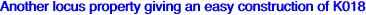Let M be a variable point on the line X(2)X(6). The parallel at X(6) to the line X(111)M meets the circle X(2)X(111)M at two points M1, M2 on K018. Similarly, the parallel at X(2) to the line X(111)M meets the circle X(6)X(111)M at two points N1, N2 on K018. These four points are concyclic on (C) and (C) is orthogonal to the circle with diameter X(2)X(6). The center Ω of (C) is the intersection of the perpendicular at M to the line X(2)X(6) and the perpendicular bisector of X(111)M. Hence Ω lies on the parabola (P) with focus X(111) and directrix the line X(2)X(6). When M traverses the line X(2)X(6), (C) envelopes a circular cubic with focus X(111) passing through X(524), X(597). This cubic meets K018 only on the line at infinity, each common point being counted three times.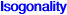K018 is invariant under isogonal conjugation with respect to ABC but also with respect to any proper triangle GPQ where G is the centroid of ABC and P, Q are two points of K018 collinear with the Lemoine point K. In particular, it is invariant under isogonal conjugation with respect to X(2)X(13)X(14) like the related K733. Other remarkable triangles are X(2)X(15)X(16) and X(2)X(6)X(111). Recall that the circumcircle of X(2)X(6)X(111) is the polar conic of the singular focus X(111). The in/excenters of any triangle GPQ lie on the axes of the Steiner ellipses which are the bisectors at G in GPQ. The roles of G and K can also be swapped and then K018 is invariant under isogonal conjugation with respect to any proper triangle KPQ where P, Q are two points of K018 collinear with the centroid G. Examples of such triangles : X(6)X(13)X(16) and X(6)X(14)X(15). The in/excenters of any triangle KPQ lie on the axes of the inconic with center K. It can be seen that two isogonal conjugates on K018 are also isogonal conjugates with respect to any of these triangles GPQ and KPQ.Miscellaneous propertiesThe polar conic of X(110) decomposes into the Brocard axis and the trilinear polar of the root X(523). It follows that the six tangents at K, X(15), X(16) and the three points on the sidelines of ABC concur at X(110). The common tangential of X(13) and X(15) is T1 and the common tangential of X(14) and X(16) is T2. These two points are collinear with the singular focus X(111). The third points on X(13)X(15) and X(14)X(16) are N1, N2 respectively also collinear with X(111). The midpoint of N1N2 is X(230) on GK. The lines T1N2 and T2N1 are parallel to the asymptote. The tangents at X(13) and X(14) met at the reflection of X(110) about G. The polar conic of X(111) is the circle through G, O, K, X(691) and naturally X(111). The tangent at X(111) to this circle meets the asymptote at X on the cubic. Recall that this asymptote is the homothetic of the axis GK under h(X111, 2). The polar conic of the point at infinity X(524) – that of the line GK – is a rectangular hyperbola passing through X(597), having one asymptote perpendicular at X(111) to GK and the other that of the cubic. *** The bisectors of the lines X(111)X(524) and X(111)X contain X(2469), X(2470) and the centers of anallagmaty E1, E2 which obviously also lie on the previous rectangular hyperbola. The cubic is then invariant under the two inversions with pole Ei which swap X(111) and Ej. *** K018 is also invariant under the Psi transformation which is the product of the reflection about one axis of the Steiner inellipse and the inversion with circle that of diameter F1F2, the foci of the ellipse. See also "Orthocorrespondence and Orthopivotal Cubics", §5 and K022. K018 is a Psi-cubic as in Table 60. Psi is the involution that swaps any point of the plane to the center of its polar conic in the McCay or Kjp cubics. In particular, Psi swaps A, B, C and the vertices of the second Brocard triangle. Hence Psi transforms the sidelines of ABC into the McCay circles. The analogous involution Psi2 introduced at K1142 also keeps K108 unchanged. Here the McCay and Kjp cubics are replaced by the stelloid K598 with radial center X(6). *** K018 is also invariant under the KW involution described in the page Table 62. See the related K185. *** K018 contains the equi-Brocard center X(368), its isogonal conjugate X(368)* and its Psi image. Recall that X(368) also lies on the Wallace hyperbola (the anticomplement of the Kiepert hyperbola) and on the three equibrocardian focal cubics K083.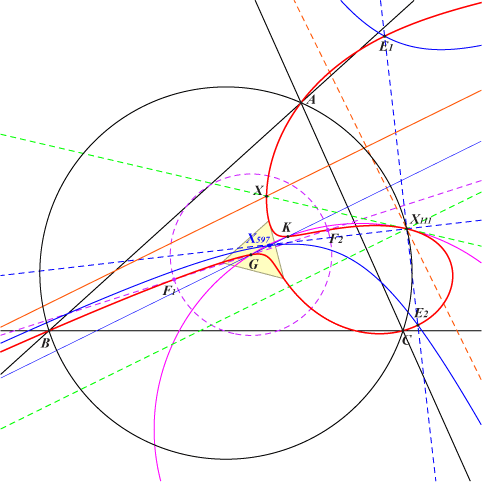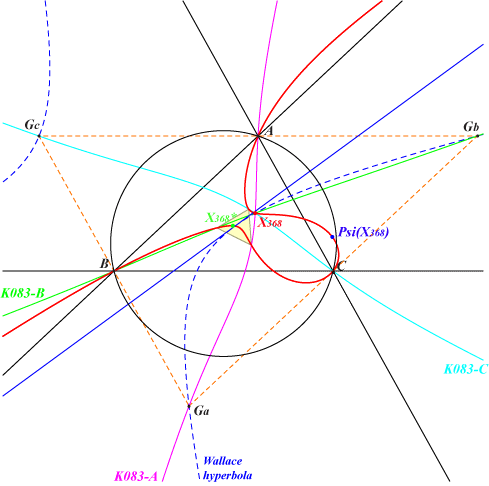The polar conic of G in K018 is a rectangular hyperbola with center K that passes through X(2), X(4), X(194), X(1689), X(1690), X(1992), X(3413), X(3414) hence it is homothetic to (K). It also contains the foci of the orthic inconic hence the tangents at these four points concur at G. Its equation is : ∑(b^2 - c^2)(SA x^2 + a^2 y z) = 0. Its Psi-image is the strophoid K1274. More generally, the polar conic of every point on the line GK is a rectangular hyperbola since GK is the orthic line of the cubic. That of K is remarkable with equation ∑(b^2 - c^2)(b^2 c^2 x^2 + 4 a^2 SA y z) = 0. Its center is G and it passes through X(3), X(6), X(381), X(599), X(2574), X(2575), hence it is homothetic to the Jerabek hyperbola. It also contains the foci of the Steiner inellipse hence the tangents at these four points concur at K. See also the strophoid K1275 for similar properties.